Analysis WebNotes
arrow_back arrow_forward

# Class Contents

## Taylor Series

### General Power Series

All of the power series that we have studied so far have been of the form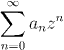and we showed that they converge inside disks centered on zero, and diverge outside those disks. However, we can easily do a change of variable, to study power series of the form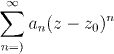where z0 is fixed. This series converges on a set of the form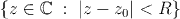which is a disk of radius R centered on z0.

In this section we shall be interested in real-valued functions of a real variable which come from power series. The power series will be of the form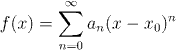where x and x0 are real numbers. The region in the real line where this series converges is the interval (x0-R, x0+R), which is called the interval of convergence (it is just the intersection of the disk of radius R centered on x0 with the real line).

### Higher Order Derivatives

In Chapter 4 we discussed functions which have a derivative at every point an interval (a,b). If f(x) is such a function, it is said to be differentiable on (a,b) and its derivative is f'.

Now it may be that the function f' is also differentiable on (a,b) and, if so, then its derivative, f'' is called the second derivative of f. By the same token, if f'' is differentiable on (a,b) then its derivative is called the third derivative of f on (a,b). In general, if we can repeat this process k times then we say that f is k-times differentiable, and that the last function obtained in this way by repeated differentiation is the kth derivative of f.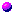Warning:

Just as there is no reason why an arbitrary function should be differentiable at all, so there is no reason, just because a function can be differentiated k times, to assume that it can be differentiated k+1 times!Notation:

We write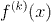for the kth derivative of f, if it exists. By convention we say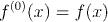### Differentiating Power Series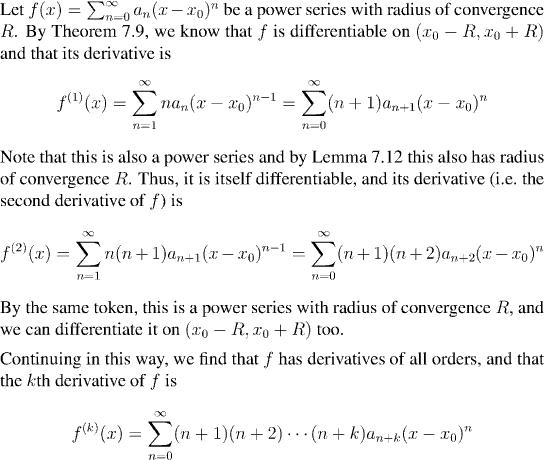References: Theorem 7.9 | Lemma 7.12

Ifis a power series, then recall that f(x0)=a0. In other words, the value of the power series at x0 is equal to the first coefficient of the series. Taking this principle to the power series we found for the derivatives of f, we find that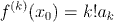Conversely,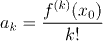Thus,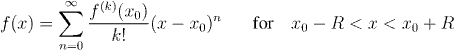We have shown that the values of the derivatives of f at just one point (namely x0) determine the values of f throughout the whole interval of convergence. When f can be expressed by a series of this kind, we call it a Taylor series.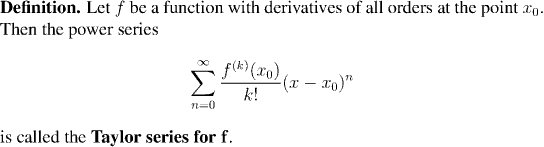We can summarize the results of the last couple of sections as: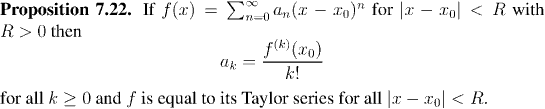It may not seem like a great revelation that f can be expressed as a power series in terms of its derivatives. After all, if we know all the derivatives of a function we already have to know a whole lot about it. There are however some very nice payoffs from the observations of the last few paragraphs.

### Power Series are Unique

A function cannot be expressed as a power series in more than one way. Put more precisely, if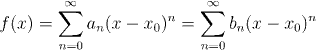for all x in a non-empty open interval, then an=bn for all n.

Question: Can you see why this is?